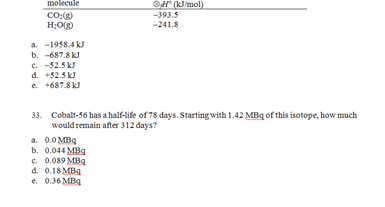### Which presents a modi solution for a transportation problem

Assignment Help Basic Statistics
##### Reference no: EM1319310

To select the non optimal solution.

Consider the following constraints from a linear programming problem.

2X + Y ≤ 200

X + 2Y ≤ 200

X,Y ≥ 0

IF these are the only constraints, which of the following points (X,Y) cannot be the optimal solution?

1.       (0,0)

2.       (0,100)

3.       (65,65)

4.       (100,0)

5.       (66.67,66.67)According to table, which presents a MODI solution for a transportation problem, if that solution is used, which of the following is true?

a.       100 units will be shipped from source A to destination 1.

b.       The total cost is \$500.

c.        The final solution is degenerate.

d.       The original problem was unbalanced.

e.        None of the above

### Write a Review